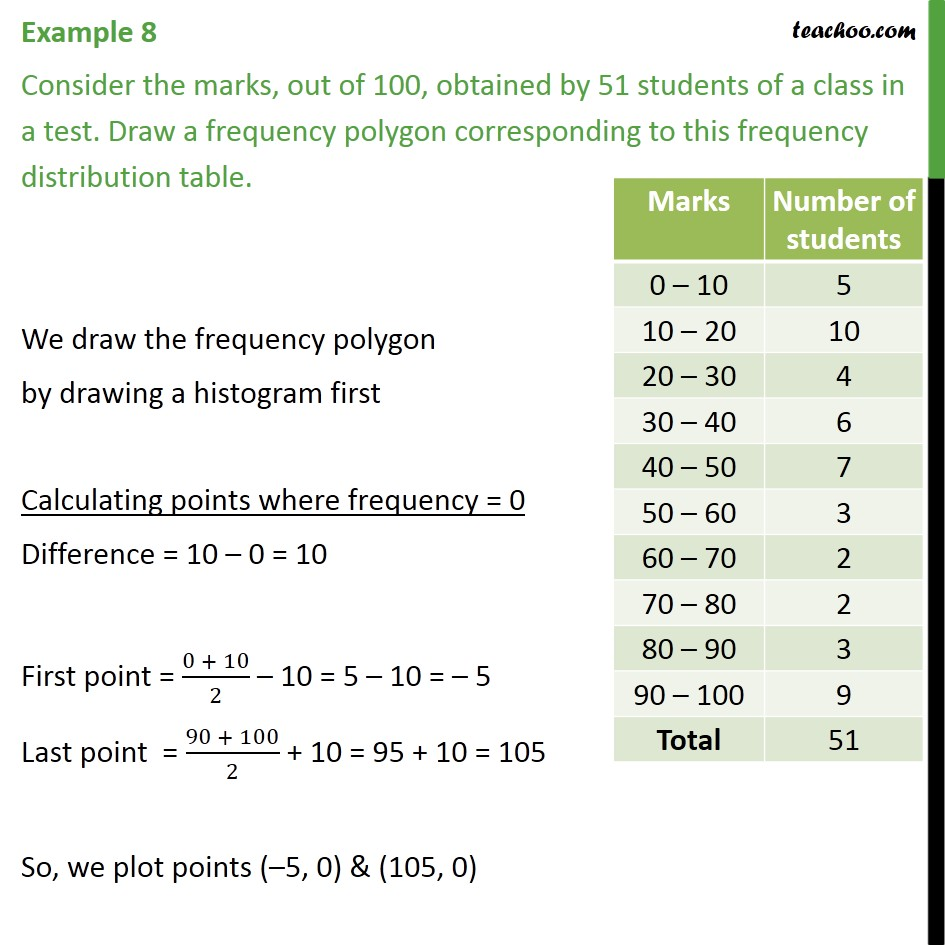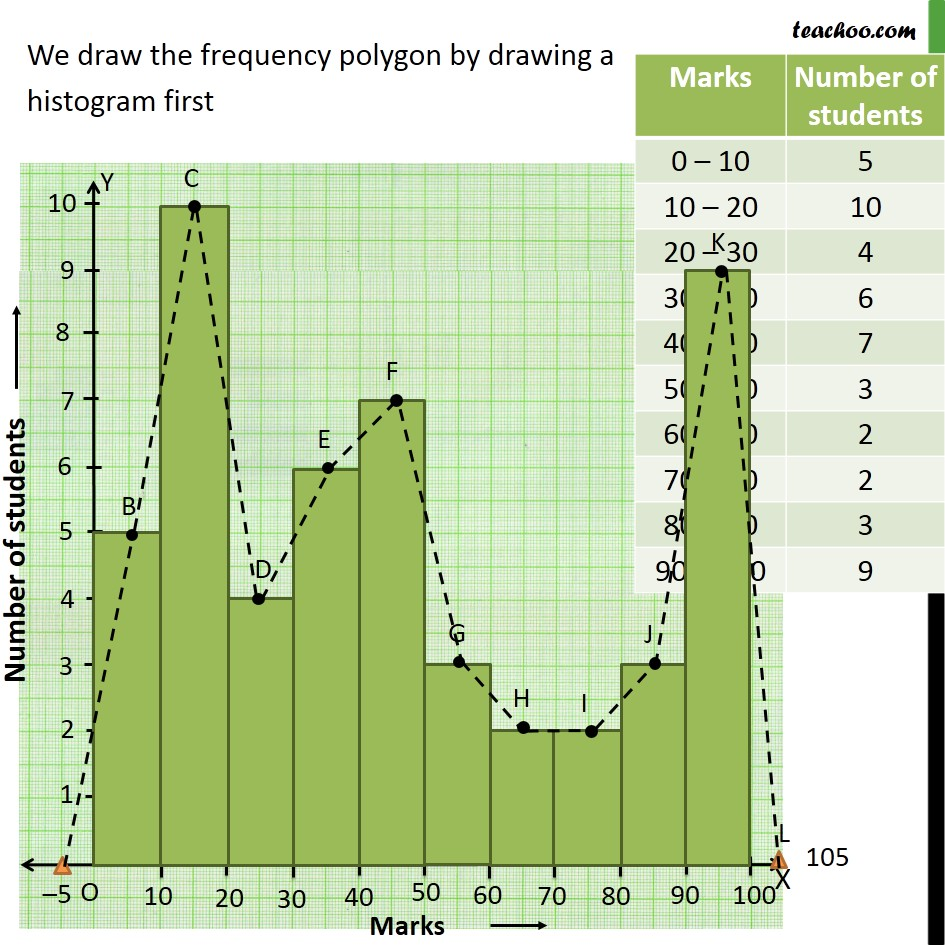Subscribe to our Youtube Channel - https://you.tube/teachoo

1. Chapter 14 Class 9 Statistics
2. Concept wise
3. Frequency polygon

Transcript

Example 8 Consider the marks, out of 100, obtained by 51 students of a class in a test. Draw a frequency polygon corresponding to this frequency distribution table. We draw the frequency polygon by drawing a histogram first Calculating points where frequency = 0 Difference = 10 0 = 10 First point = (0 + 10)/2 10 = 5 10 = 5 Last point = (90 + 100)/2 + 10 = 95 + 10 = 105 So, we plot points ( 5, 0) & (105, 0)

Frequency polygon

About the AuthorDavneet Singh
Davneet Singh is a graduate from Indian Institute of Technology, Kanpur. He has been teaching from the past 9 years. He provides courses for Maths and Science at Teachoo.